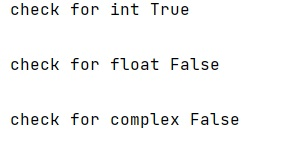# How to check if a NumPy dtype is integral?

Learn, how to check if a NumPy dtype is integral?
Submitted by Pranit Sharma, on March 14, 2023

## Checking whether a NumPy dtype is integral or not?

Data types are the category of values or types of values which can be used in programming for computations. To store different types of values (integer, decimal, characters, etc.) we need different data types in Python or any other programming language(s).

Numpy has a hierarchy of dtypes similar to a class hierarchy (the scalar types have a bona fide class hierarchy that mirrors the dtype hierarchy). We can use numpy.issubdtype(some_dtype, numpy.integer) to test if a dtype is an integer dtype.

Let us understand with the help of an example,

## Python code to check if a NumPy dtype is integral

```# Import numpy
import numpy as np

# Checking for different data types
print("check for int",np.issubdtype(np.int32, np.integer),"\n")
print("check for float",np.issubdtype(np.float32, np.integer),"\n")
print("check for complex",np.issubdtype(np.complex64, np.integer),"\n")
```

### Output